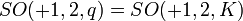# Split special orthogonal group of degree two

## Definition

Suppose$K$ is a field. The split special orthogonal group of degree two over$K$ is defined as the subgroup of the general linear group of degree two over$K$ given as follows:$\{ A \in GL(2,K) \mid \operatorname{det}(A) = 1, A\begin{pmatrix} 0 & 1 \\ 1 & 0 \\\end{pmatrix}A^T = \begin{pmatrix} 0 & 1 \\ 1 & 0 \\\end{pmatrix}\}$

For characteristic not equal to two, an alternative definition, which gives a conjugate subgroup and hence an isomorphic group, is:$\{ A \in GL(2,K) \mid \operatorname{det}(A) = 1, A\begin{pmatrix} 1 & 0 \\ 0 & -1 \\\end{pmatrix}A^T = \begin{pmatrix} 1 & 0 \\ 0 & -1 \\\end{pmatrix} \}$

### Over a finite field

For a finite field$K$, this group is denoted$SO(+1,2,K)$ and is termed the orthogonal group of "+" type. It is also denoted$SO(+1,2,q)$ where$q$ is the size of the field.

It turns out that:

• If$q$ is a power of 2, i.e., if$q$ is even, then the group is a dihedral group of order$2(q - 1)$ and degree$q - 1$.
• If$q$ is odd, then the group is a cyclic group of order$q - 1$.

## Arithmetic functions

### Over a finite field

We consider here the group$SO(+1,2,q) = SO(+1,2,K)$ where$K$ is a field (unique up to isomorphism) of size$q$.

Function Value Similar groups Explanation
order Case$q$ odd:$q - 1$
Case$q$ even:$2(q - 1)$

## Particular cases$q$ (field size)$p$ (underlying prime, field characteristic) exponent on$p$ giving$q$ special orthogonal group$SO(+1,2,q)$ order of group second part of GAP ID (GAP ID is (order,2nd part) Comments
2 2 1 cyclic group:Z2 2 1
3 3 1 cyclic group:Z2 2 1
4 2 2 symmetric group:S3 6 1
5 5 1 cyclic group:Z4 4 1
7 7 1 cyclic group:Z6 6 2
8 2 3 dihedral group:D14 14 1
9 3 2 cyclic group:Z8 8 1
11 11 1 cyclic group:Z10 10 2
13 13 1 cyclic group:Z12 12 2
16 2 4 dihedral group:D30 30 3
17 17 1 cyclic group:Z16 16 1